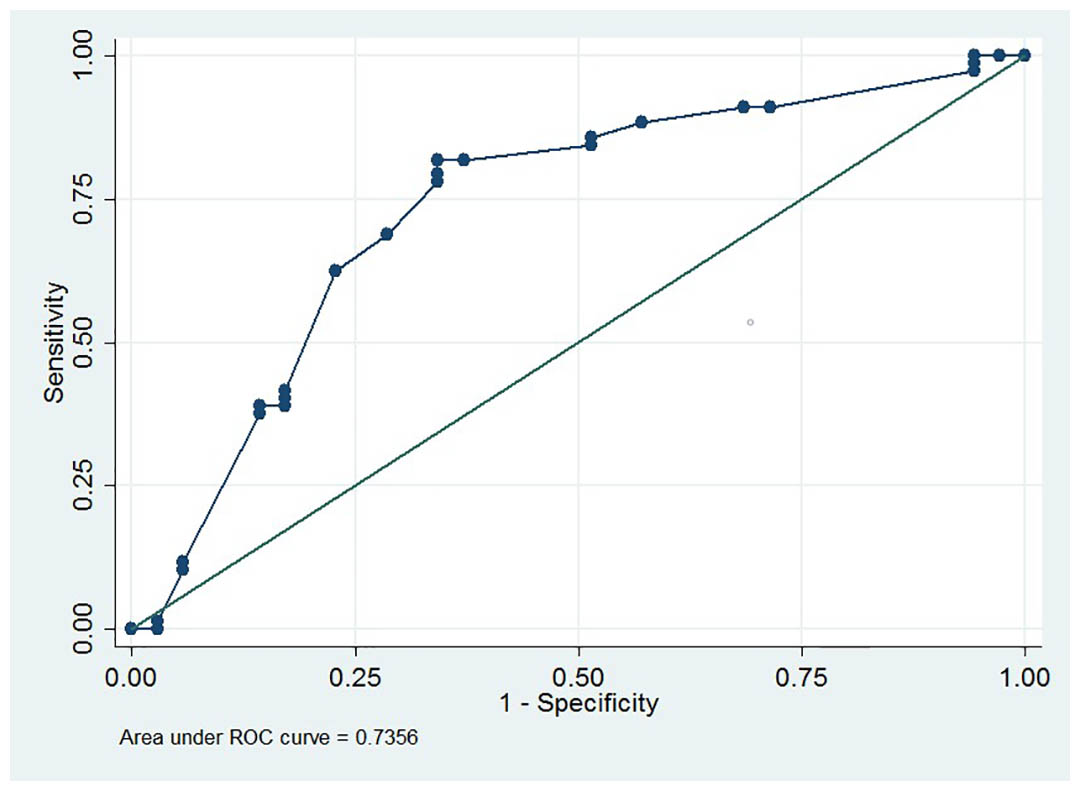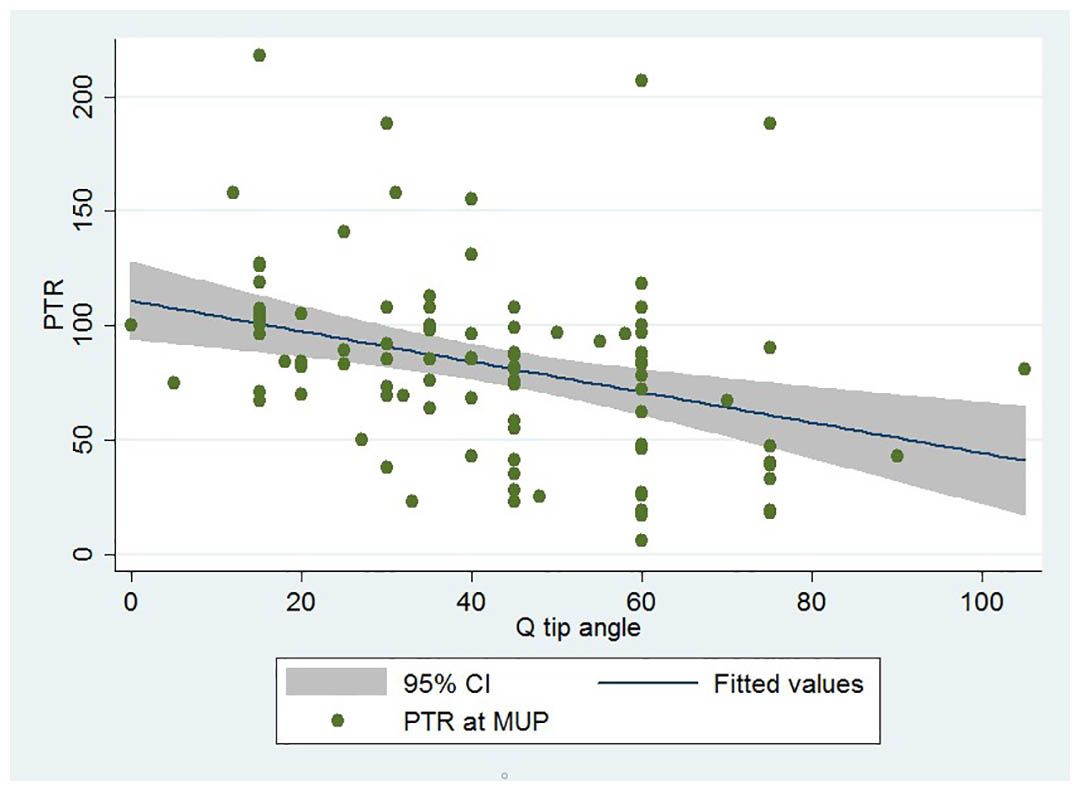Hsiao S1, Lin H2

Research Type

Clinical

Abstract Category

Urodynamics

 Links Abstract 173 E-Poster 1 Scientific Open Discussion ePoster Session 7 Wednesday 4th September 2019 12:55 - 13:00 (ePoster Station 11) Exhibition Hall Incontinence Female Urodynamics Techniques Stress Urinary Incontinence 1.Department of Obstetrics and Gynecology, Far Eastern Memorial Hospital, New Taipei, Taiwan, 2.Department of Obstetrics and Gynecology, National Taiwan University College of Medicine and National Taiwan University Hospital, Taipei, Taiwan Presenter S Sheng-Mou Hsiao

Abstract

Hypothesis / aims of study
Urethral hypermobility, which can be assessed with Q-tip test, has been considered to be partly responsible for pathogenesis of women with stress urinary incontinence (SUI). Nonetheless, Q-tip test has lost favor due to patient discomfort. Thus, the main objective of this study is to identify clinical or urodynamic predictors of the Q-tip angle. The secondary objective of this study is to identify a clinical or urodynamic surrogate for the Q-tip test.
Study design, materials and methods
Between January 2014 and September 2018, 112 consecutive women with lower urinary tract symptoms who underwent Q-tip testing and urodynamic studies were included in this retrospective study.
The Q-tip angle was defined as the maximum straining angle from the horizontal plane. A Q-tip angle ≥ 30 degrees was defined as indicating urethral hypermobility.
STATA software (Version 11.0; Stata Corporation, College Station, Texas, USA) was used for statistical analysis. Spearman’s rank correlation coefficient and multivariable backward stepwise linear regression analysis were performed as appropriate. Multivariable backward stepwise linear regression analysis was performed on all significant variables in Spearman’s rank correlation coefficient to identify predictors of the Q-tip angle. A p value <0.05 was considered statistically significant.
Results
A total of 112 women were reviewed in the study.The average Q-tip angle was 41.8 degrees. Based on the receiver operating characteristic curve (ROC) analysis, the optimum cut-off value of the Q-tip angle was ≥ 32 degrees to predict urodynamic stress incontinence (USI) and was determined with the area under the ROC curve being 0.74 (95% confidence interval [CI] = 0.63 to 0.84; sensitivity = 81.8%, specificity = 65.7%, Fig 1).
Age, point Aa, point Ba, point C, USI, detrusor overactivity, pad weight, maximum flow rate, voided volume, functional profile length and pressure transmission ratio (PTR) at maximum urethral pressure (MUP) were significantly correlated with the Q-tip angle.
Multivariable linear regression analysis revealed that point Aa (a, coefficient = 4.8), urodynamic stress incontinence (USI, b, coefficient = 11.7), detrusor overactivity (c, coefficient = -11.3), maximum flow rate (d, coefficient = 0.13) and PTR at MUP (e, coefficient = -0.16) were independent predictors of the Q-tip angle with a constant of 53.8. The predicted Q-tip angle can be denoted by Q-tip angle = 53.8 + 4.8 x a + 11.7 x b – 11.3 x c + 0.13 x d - 0.16 x e.
PTR at MUP (rho = -0.41, Fig 2) had a higher correlation with the Q-tip angle compared with point Aa (rho = 0.38), USI (rho = 0.38), detrusor overactivity (rho = -0.29) and maximum flow rate (rho = 0.26).
PTR at MUP was different between USI and non-USI women. Based on the ROC analysis, the optimum cut-off values of PTR at MUP < 81% to predict USI was determined with the area under the ROC curve being 0.74 (95% CI = 0.64 to 0.83; sensitivity = 85.7%, specificity = 54.9%).
If performing univariate regression analysis with the Q-tip angle, the coefficient of PTR at MUP was -0.18 (95% CI = -0.27 to -0.08, p < 0.001) with a constant of 56.7 (95% CI = 48.1 to 65.4, p < 0.001). Thus, the predicted Q-tip angle for a given PTR at MUP (%, a) could be denoted by Q-tip angle = 56.7 - 0.18 x a. R2 was 0.12.
In addition, a significant correlation between PTR at MUP (< 81 vs. ≥ 81%) and the Q-tip angle (≥ 30 vs. < 30 degrees) was found (rho = -0.29, p = 0.003). Based on the ROC analysis to predict USI, the optimum cut-off value of PTR at MUP < 81% was determined with the ROC area of 0.74.
Interpretation of results
Our data shows that a good correlation between urethral hypermobility and pressure transmission ratio. However, some factors, such as point Aa and detrusor overactivity, can affect Q-tip angle, not only pressure transmission ratio.
Concluding message
Point Aa, USI, detrusor overactivity, maximum flow rate and PTR at MUP were independent predictors for the Q-tip angle. In addition, PTR at MUP might be used as a surrogate for Q-tip testing.
Figure 1Figure 2Disclosures
Funding none Clinical Trial No Subjects Human Ethics Committee Far Eastern Memorial Hospital Research Ethics Review Committee Helsinki Yes Informed Consent No